Web www.handwritingforkids.com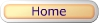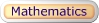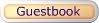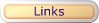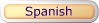### Online Math 2 - Subtraction Math Drill/Test (Horizontal)

This online/interactive generator will provides plenty of math drill and practice in subtraction, to boost test scores, improves accuracy and speed to prepare the child/children to meet the Kindergarten, Grade 1, and Grade 2 curriculum standards. This online/interactive math addition, will create 1 digit by 1 digit (1x1) equations with an option of timed test, and it will automatically graded/scored your results in a printable worksheet.

minuend
subtrahend
difference8 - 4 = 4

 Type of online math problems: Online Math 2 - Subtraction - Difference 0 to 5 Online Math 2 - Subtraction - Difference 5 to 10 Online Math 2 - Subtraction - Difference 0 to 10 Online Math 2 - Subtraction - Difference 0 to 18 Online Math 2 - Subtraction - Addition: 10 to 18

2. Select the number of math problems per page. (Default: 1 - 18)
3. Select Type of Math Problems: (Default: No Carryovers)
4. Do you want a timed-test? (Default: Yes)
5. Click on submit to make your personalized online horizontal math subtraction drill/test.
6. Option: Page back and click Submit to create a different set of math problems.

Online Math 2 - Subtraction Math Drill Maker (Horizontal)

 Name: (optional)

Enter the Number of Math Problems per page:

Default: 1 - 18

Type of Math Problems:

Default: Difference 0 to 10

Yes, Timed-TestBasic Handwriting for Kids Free for Non-Profit Use Ms. Readman, Rockford, IL Revised: